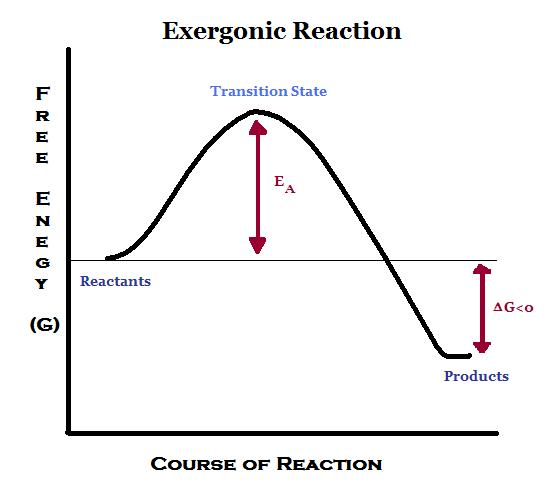# Difference Between Endergonic and Exergonic

## Main Difference – Endergonic vs Exergonic

The terms endergonic and exergonic are used to explain two types of chemical reactions. An endergonic reaction is a non-spontaneous reaction. It does not occur in normal conditions such as at room temperature and atmospheric pressure. An exergonic reaction is the opposite of endergonic reaction. An exergonic reaction is a spontaneous reaction. It occurs at normal conditions without any external force. Each and every chemical reaction can be categorized as an endergonic reaction or an exergonic reaction. The main difference between endergonic and exergonic is that endergonic reaction requires energy from the outside whereas exergonic reactions release energy to the outside.

### Key Areas Covered

1. What is Endergonic
– Definition, Explanation with Thermodynamics
2. What is Exergonic
– Definition, Explanation with Thermodynamics
3. What is the Difference Between Endergonic and Exergonic
– Comparison of Key Differences

Key Terms: Endergonic, Enthalpy, Entropy, Exergonic, Gibbs Free Energy, Spontaneous Reaction## What is Endergonic

Endergonic is a type of reaction that has a positive Gibbs free energy. The Gibbs free energy is a thermodynamic potential that is used to predict whether a chemical reaction is spontaneous or non-spontaneous. A negative Gibbs free energy indicates a spontaneous reaction. In case of endergonic reactions, the Gibbs free energy is a positive value, which indicates it is a no-spontaneous reaction. Non-spontaneous reactions can also be named as unfavorable reactions.

The Gibbs free energy of endergonic reaction is a positive value when calculated using the following thermodynamic relationship.

ΔG   =    ΔH  –  TΔS

Where,  ΔG is the Gibbs free energy

ΔH is the change in enthalpy

T is the temperature of the system

ΔS is the change in entropy.Figure 1: Energy Diagram for an Endergonic Reaction

In a non-spontaneous reaction, energy should be provided from outside for the progression of the reaction. Then, the energy of the products gets a higher value than that of the energy of the reactants. Due to that reason, the change in enthalpy is a positive value (the change in the enthalpy is the difference between the enthalpies of products and reactants). Since new products are formed, the entropy of the system is decreased. Then, according to the above equation, the ΔG is a positive value. Endergonic reactions include endothermic reactions.

## What is Exergonic

Exergonic is a type of reaction that has a negative Gibbs free energy. This indicates that an exergonic reaction is a spontaneous reaction since spontaneous reactions have a negative value for Gibbs energy when calculated using the thermodynamic relationship given below.

ΔG    =    ΔH  –  TΔSFigure 1: Energy Diagram for an Exgorgenic Reaction

In exergonic reactions, energy is released to the surrounding. Therefore, the products have a lower energy than that of the reactants. Due to that reason, the change in enthalpy is a negative value for exergonic reactions. The entropy is increased due to the disorder of the system. According to the above relationship, the Gibbs free energy is a negative value. Exergonic reactions include exothermic reactions.

## Difference Between Endergonic and Exergonic

### Definition

Endergonic: Endergonic is a type of reaction that has a positive Gibbs free energy.

Exergonic: Exergonic is a type of reaction that has a negative Gibbs free energy.

### Gibbs Free Energy

Endergonic: The Gibbs free energy is a positive value for endergonic reactions.

Exergonic: The Gibbs free energy is a negative value for exergonic reactions.

### Energy of Reactants and Products

Endergonic: In endergonic reactions, the energy of reactants is lower than that of the products.

Exergonic: In exergonic reactions, the energy of reactants is higher than that of the products.

### Entropy

Endergonic: The entropy is decreased in endergonic reactions.

Exergonic: The entropy is increased in exergonic reactions.

### Nature

Endergonic: Endergonic reactions are non-spontaneous.

Exergonic: Exergonic reactions are spontaneous.

### Examples

Endergonic: Endothermic reactions are endergonic reactions.

Exergonic: Exothermic reactions are exergonic reactions.

### Beginning of the Reaction

Endergonic: Endergonic reactions always require energy to begin the reaction.

Exergonic: Exergonic reactions do not need energy to begin the reaction.

### Energy Exchange

Endergonic: Endergonic reactions absorb energy from the surrounding.

Exergonic: Exergonic reactions release energy to the surrounding.

### Conclusion

All chemical reactions that happen in our surrounding can be categorized as either endergonic reaction or exergonic reactions. These two types of reactions have opposite definitions and properties. The main difference between endergonic and exergonic is that endergonic reaction requires energy from outside whereas exergonic reactions release energy to the outside.

##### References:

1. “Endergonic Reaction: Definition & Examples.” Study.com, Available here. Accessed 21 Sept. 2017.
2. Helmenstine, Ph.D. Anne Marie. “Understand the Difference Between Endergonic and Exergonic.” ThoughtCo, Available here. Accessed 21 Sept. 2017.
3. “Endergonic reaction.” Wikipedia, Wikimedia Foundation, 1 Sept. 2017, Available here. Accessed 21 Sept. 2017

##### Image Courtesy:

1.”Endergonic” By J3hoang – Own work (CC BY-SA 3.0) via Commons Wikimedia
2.”Exergonic” By J3hoang – Own work (CC BY-SA 3.0) via Commons Wikimedia